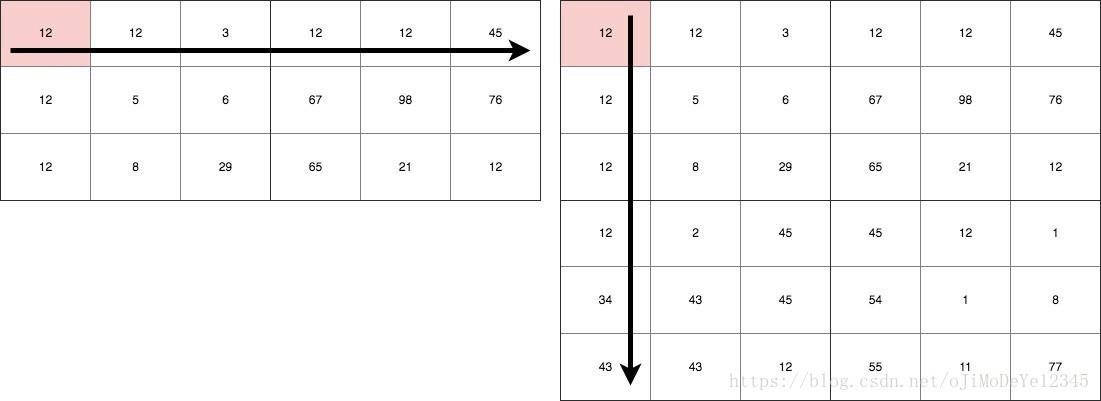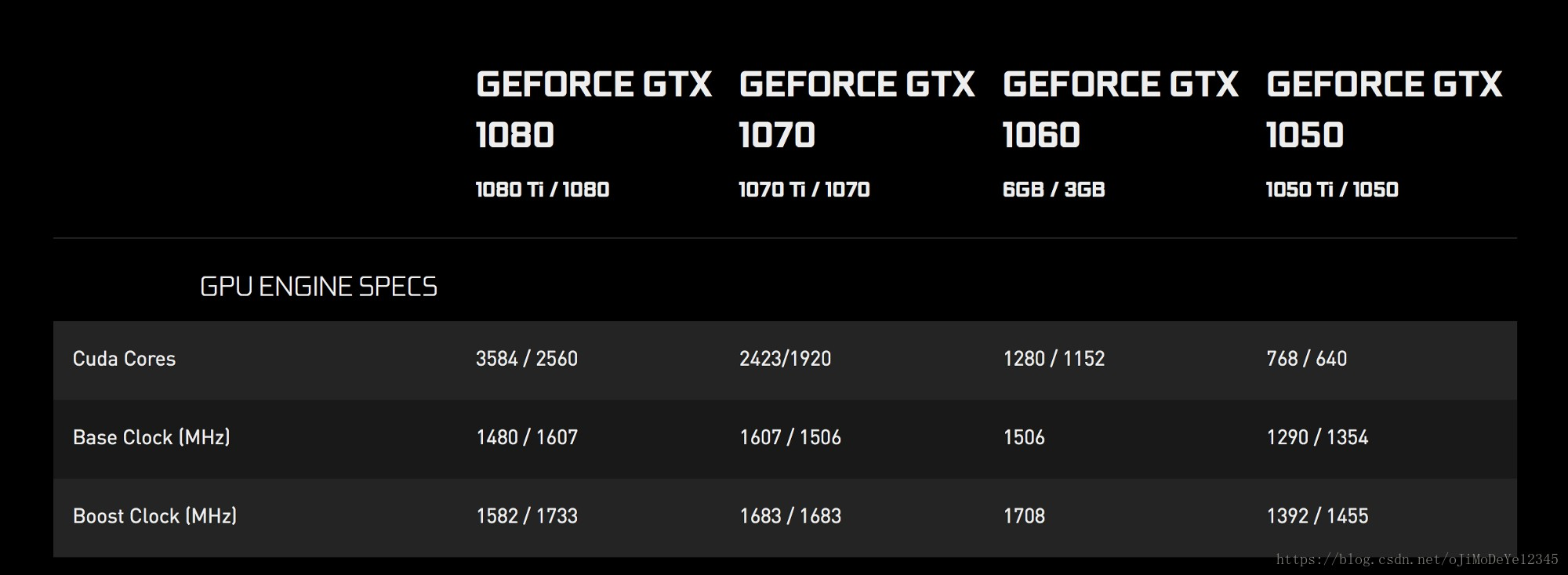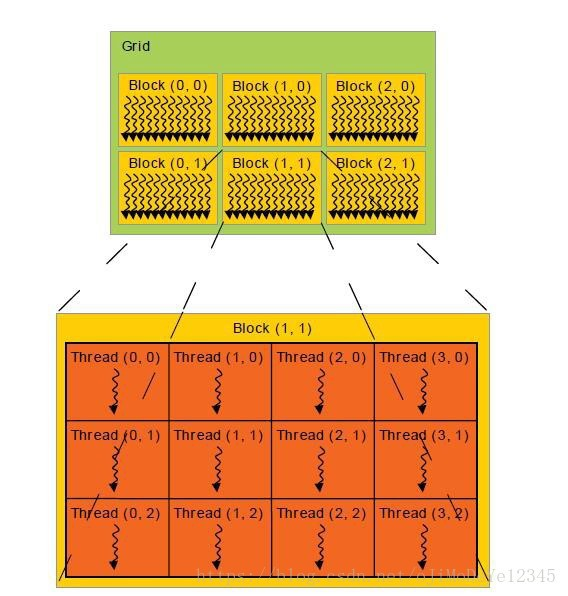GPU 优化简介 原文 - An Introduction to GPU Optimization

<h2>矩阵乘法 Matrix Multiplication</h2>Figure 1. 矩阵乘法

<h2>GPUs 简介</h2>

GPUs 通常包含大量的处理核心(processing cores). 数量一般从 384 到上千个. 如图，NVIDIA 的部分显卡 GPUs.Figure 2. NVIDIA GPUs CUDA 核心数

CUDA，Compute Unified Device Architecture. 其运行速度运行相对较慢，但通过利用大量的ALUs(Arithmetic and Logical Units)，具有强大的并行化能力. 可参见 WHAT IS GPU-ACCELERATED COMPUTING?<h2>关于 GPU 的思考</h2>

<h2>基于 APARAPI 的 GPU 编程</h2>

APARAPI (A-PARallel-API) 是 OpenCL 的 JAVA 接口，是用于 GPUs 编程的开放式计算语言，其支持 CUDA 结构和 AMD 设备.

• pom.xml
<?xml version="1.0" encoding="UTF-8"?>
<project xmlns="http://maven.apache.org/POM/4.0.0"
xmlns:xsi="http://www.w3.org/2001/XMLSchema-instance"
xsi:schemaLocation="http://maven.apache.org/POM/4.0.0 http://maven.apache.org/xsd/maven-4.0.0.xsd">
<modelVersion>4.0.0</modelVersion>

<groupId>cuda-aparapi</groupId>
<artifactId>cuda</artifactId>
<version>1.0-SNAPSHOT</version>

<dependencies>
<dependency>
<groupId>com.aparapi</groupId>
<artifactId>aparapi</artifactId>
<version>1.4.1</version>
</dependency>
</dependencies>

</project>

</li> <li>MatrixMultiplication.java
import com.aparapi.Kernel;
import com.aparapi.Range;

/**
* Created by anuradhawick on 12/29/17.
*/
public class MatrixMultiplication {

public static void main(String[] args) {
// Width of the matrix
final int SIZE = 5000;

// We should use linear arrays as supported by the API
final int[] a = new int[SIZE * SIZE];
final int[] b = new int[SIZE * SIZE];
int[] c = new int[SIZE * SIZE];
final int[] d = new int[SIZE * SIZE];
int val;

// Creating random matrices
for (int i = 0; i < SIZE; i++) {
for (int j = 0; j < SIZE; j++) {
a[i  SIZE + j] = (int) (Math.random()  100);
b[i  SIZE + j] = (int) (Math.random()  100);
}
}
long time = System.currentTimeMillis();

// CPU multiplication
for (int i = 0; i < SIZE; i++) {
for (int j = 0; j < SIZE; j++) {
val = 0;
for (int k = 0; k < SIZE; k++) {
val += a[i  SIZE + k]  b[k * SIZE + j];
}
c[i * SIZE + j] = val;
}
}
System.out.println("Task finished in " + (System.currentTimeMillis() - time) + "ms");

// Kernel for multiplication
Kernel kernel = new Kernel() {
@Override
public void run() {
int row = getGlobalId() / SIZE;
int col = getGlobalId() % SIZE;
if (row > SIZE || col > SIZE) return;

d[row * SIZE + col] = 0;

for (int i = 0; i < SIZE; i++) {
d[row  SIZE + col] += a[row  SIZE + i]  b[i  SIZE + col];
}
}
};

// Array size for GPU to know
Range range = Range.create(SIZE * SIZE);

System.out.println("Starting GPU computation");
time = System.currentTimeMillis();
kernel.execute(range); // Running the Kernel
System.out.println("Task finished in " + (System.currentTimeMillis() - time) + "ms");

// Verifying the result
for (int i = 0; i < SIZE; i++) {
for (int j = 0; j < SIZE; j++) {
if (c[i  SIZE + j] != d[i  SIZE + j]) {
System.out.println("ERROR");
return;
}
}
}
}
}

</li> </ul> 核Kernel是 GPU 执行代码的一部分， Kernel 可见的变量被复制到 GPU RAM. 以上代码中，GPUs 组织数据的形式是线性数组(linear array)，而非 2D 数组的形式. 虽然，GPUs 不能处理 2D 数组，但其数据处理方式类似于数组维度.
Range range = Range.create(SIZE * SIZE);


分配 GPU 内存，GPU 最多会运行 SIZE × SIZE 个线程.

int row = getGlobalId() / SIZE;
int col = getGlobalId() % SIZE;


从线程的专有内存(private memory) 获取线程 Id. 根据 Id 即可确定线程的单元格位置.

对于每个单元格，计算

for (int i = 0; i < SIZE; i++) {
d[row  SIZE + col] += a[row  SIZE + i]  b[i  SIZE + col];
}


仅仅是对两个矩阵对应单元格的计算结果进行相加.

只需采用线程索引定义单个线程的 Kernel. 所有线程并行化运行.

GPU 计算的速度会有多快呢，以上代码的输出为：

// 1200 x 1200
Starting GPU computation


相差很大！

由于 CPU 耗时较久，以下仅为 GPU 计算耗时：

// 2000 x 2000